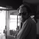12921 lượt xem
12921
This is an experimental Indicator based on Momentum. Picks the Top & Bottom Candle for most Swing. Please post your opinion or suggestion to improve this indicator.

I have not back tested this completely. This is recently developed Indicator.

//Short Term Top and Bottom Candle Identifier
//Code by UCSgears

study("UCS_Top & Bottom Candle", shorttitle = "UCS_T&B", overlay=false)

a = input(5, "Percent K Length")
b = input(3, "Percent D Length")

// Range Calculation
ll = lowest (low, a)
hh = highest (high, a)
diff = hh - ll
rdiff = close - (hh+ll)/2

// Nested Moving Average for smoother curves
avgrel = ema(ema(rdiff,b),b)
avgdiff = ema(ema(diff,b),b)

// Momentum
mom = ((close - close[b])/close[b])*1000

// SMI calculations
SMI = avgdiff != 0 ? (avgrel/(avgdiff/2)*100) : 0
SMIsignal = ema(SMI,b)

//All PLOTS
plot(mom, title = "Momentum", style = columns, color = yellow)
plot(SMI, title = "Stochastic Momentum Index")
plot(SMIsignal, color= red, title = "SMI Signal Line")

H1 = hline(40, color = red, title = "Over Bought")
H2 = hline(-40, color = green, title = "Over Sold")
H0 = hline(0, color = blue, title = "Zero Line")
fill(H0,H2, color = green, title = "Oversold")
fill(H0,H1, color = red, title = "Overbought")
//END

// Strategy Signals
barcolor(short)